2022: SklogWiki celebrates 15 years on-line

# Ideal gas Helmholtz energy function

From equations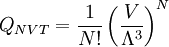$Q_{NVT}=\frac{1}{N!} \left( \frac{V}{\Lambda^{3}}\right)^N$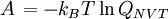$\left.A\right.=-k_B T \ln Q_{NVT}$

for the Helmholtz energy function, one has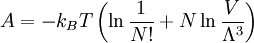$A=-k_BT\left(\ln \frac{1}{N!} + N\ln\frac{V}{\Lambda^{3}}\right)$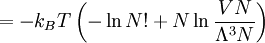$=-k_BT\left(-\ln N! + N\ln\frac{VN}{\Lambda^3N}\right)$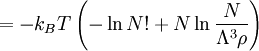$=-k_BT\left(-\ln N! + N\ln\frac{N}{\Lambda^3 \rho}\right)$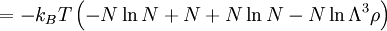$=-k_BT\left( -N\ln N +N + N\ln N - N\ln \Lambda^3 \rho \right)$

one arrives at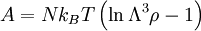$A=Nk_BT\left(\ln \Lambda^3 \rho -1 \right)$

where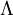$\Lambda$is the de Broglie thermal wavelength and$k_B$ is the Boltzmann constant.